•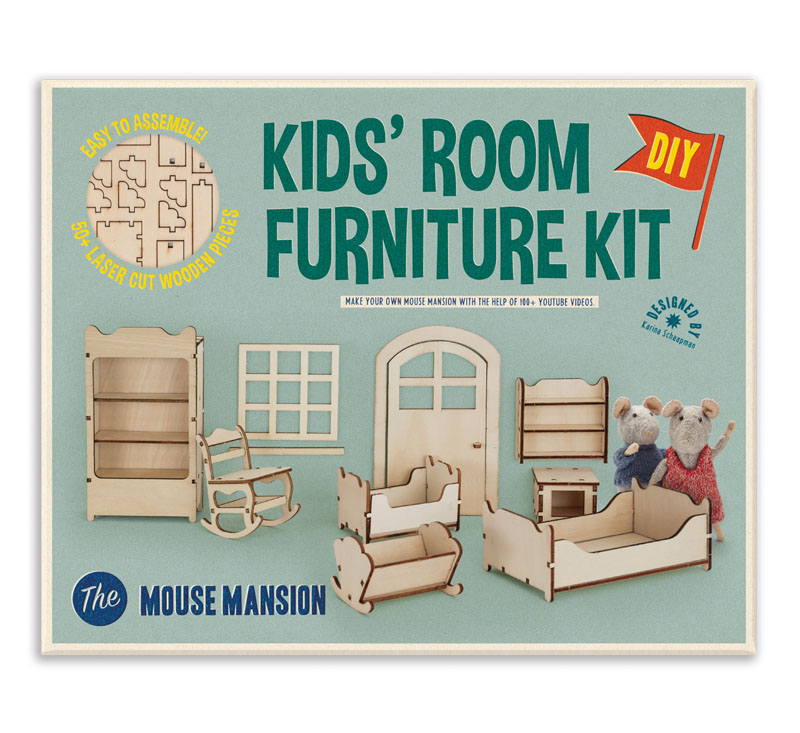•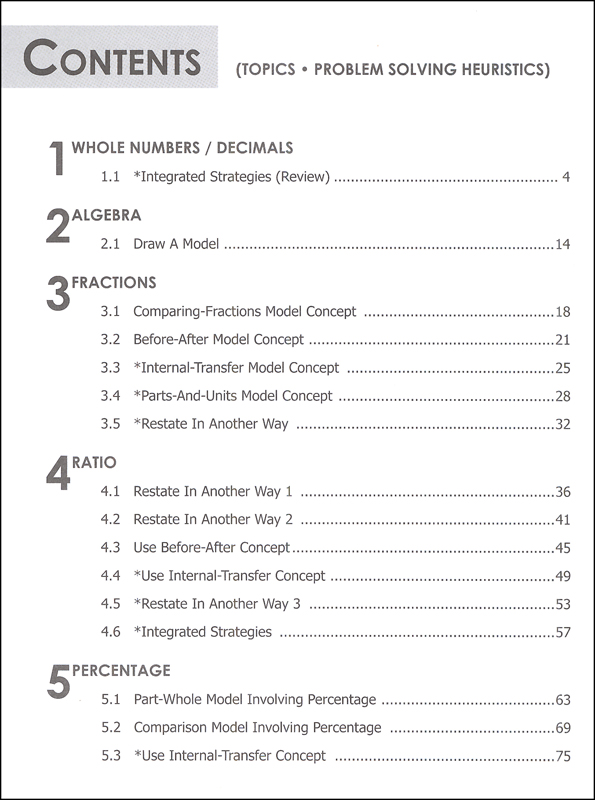•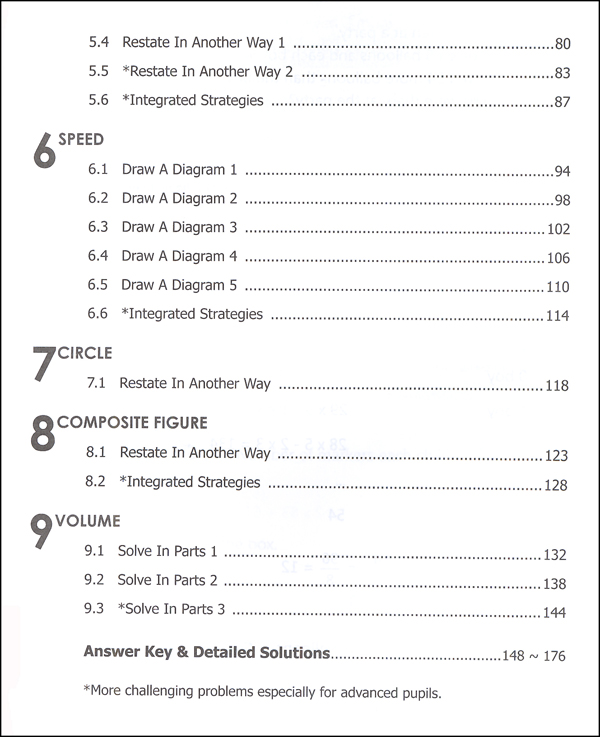•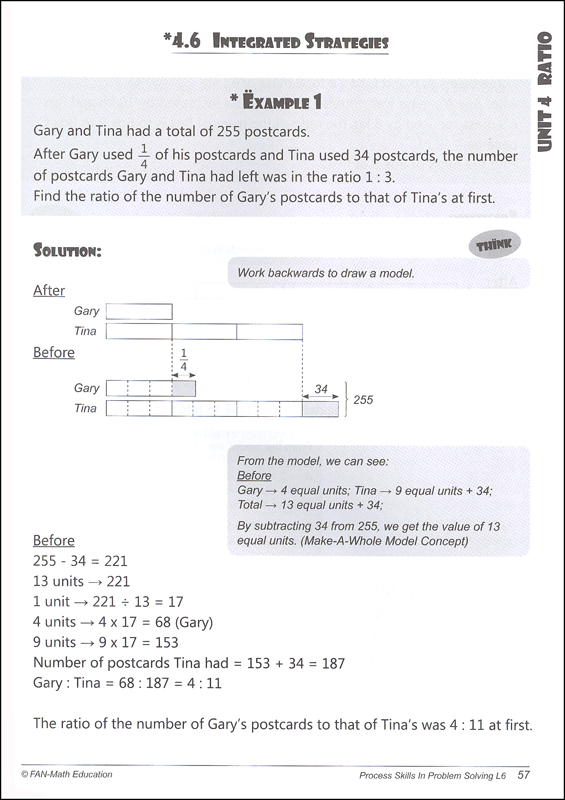•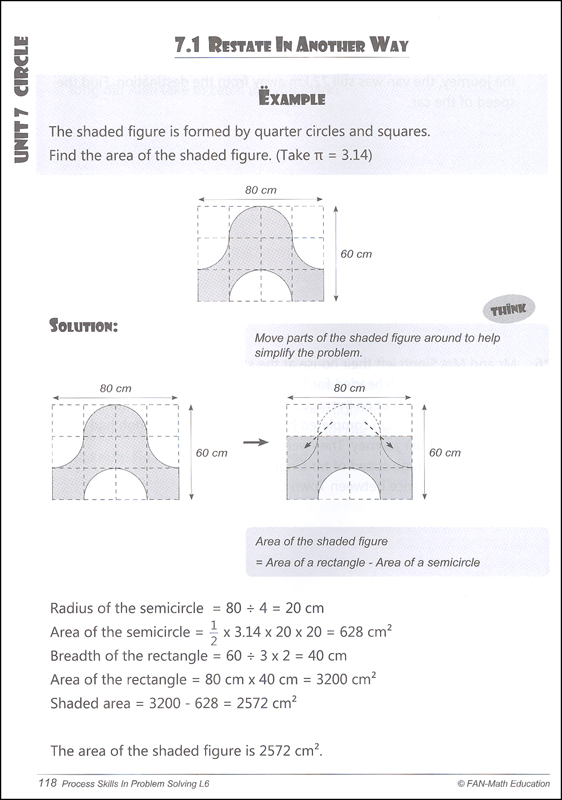# Process Skills in Problem Solving Level 6

# 003976

Our Price: \$14.80
2 In Stock. Item will be unavailable when sold out.
Qty:
Qty:

 Item #: 003976 9789810721008 6

Category Description for Process Skills in Problem Solving:

This series comes from the writer of Top 10 Maths, Li Fanglan. His approach is heuristic (discoveries resulting from investigations made by the student) encouraging students to think symbolically (Bar Model Approach.) Using the Singapore approach to teach kids to think mathematically, these workbooks provide practice in the following areas. Each book for Levels 1 through 3 is divided into two sections. The first section uses the Model Approach (MA), followed by a section using the Heuristic Approach (HA). Levels 4 and up focus on Heuristic approach methods. I am listing the model concepts because they seem important to the methodology, but be sure to look at the topics listed in each level.

Level 1: (MA) Addition/subtraction (within 10, comparisons, within 40. (HA) systematic listing, patterns with shapes/numbers, draw diagram, restate problem, guess & check. 102pp

Level 2: (MA) Addition/subtraction (within 1000, involving comparison, 2-step word problems.) Multiply/divide (by 2, 3, 4, 5 and 10, 4 operations.) (HA) systematic listing I, work backwards, look for patterns, restate problem, draw diagram, systematic listing II, spatial visualization, combined strategies. 101pp

Level 3: (MA) Addition (part-whole model, 1-step routine word problems, equal concept 1-2-3, comparison model, 1-step routine word problems, comparison concept I-II, difference concept.) Subtraction (2-step word problems.) Multiplication (model drawing, 1-step word problems, multiple concept, 2-step word problems.) Division (model drawing, 1-step word problems, multiple concepts, 2-step word problems.) Four operations (challenging word problems.) (HA) act it out, look for patterns, work backwards, draw diagram, restate problem, make supposition, combined strategies. 120pp

Level 4: (HA) Addition/subtraction (review.) Then they go over multiplication/division of whole numbers before getting into fractions (add/subtract, unit model concept, mixed problems.) Decimals (add/subtract/multiply/divide.) Area/perimeter (restate, systematic listing.) 128pp

Level 5: (HA) Whole numbers (pattern, listing, parts, make-a-whole, before-after, internal-transfer, restate, integrated strategies.) Fractions (simplify calculation, part-whole, comparison, parts-units, restate, before-after, parts, parts-units, work backwards. Ratio (unit model, before-after, restate, draw diagram, before-after concepts I-II.) Decimals (draw model, restate.) Percentage of a Quantity (part-whole concepts, comparison, before-after.) Average (solve in parts, restate.) Area of Triangle (restate.) Volume (solve in parts.) 176pp

Level 6: (HA) Whole numbers/decimals (review.) Algebra (draw a model.) Fractions (comparing, before-after, internal transfer, parts-units, restate.) Ratio (restate 3 ways, before-after, internal transfer, integrated strategies.) Percentage (part-whole, comparison, internal-transfer, restate 2 ways, integrated strategies.) Speed (draw 5 diagrams, integrated strategies.) Circle (restate.) Composite Figure (restate, integrated strategies.) Volume (solve in 3 parts.) 176pp

Answer keys with detailed solutions are in the back of each book. pb ~ Sara

Primary Subject
Mathematics
6
ISBN
9789810721008
Format
Softcover Book
Brand Name
SingaporeMath.com
Weight
0.7 (lbs.)
Dimensions
10.25" x 7.5" x 0.25"